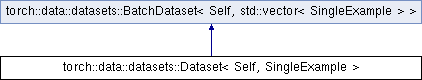Caffe2 - C++ API A deep learning, cross platform ML framework
torch::data::datasets::Dataset< Self, SingleExample > Class Template Referenceabstract

A dataset that can yield data in batches, or as individual examples. More...

`#include <base.h>`

Inheritance diagram for torch::data::datasets::Dataset< Self, SingleExample >:## Public Types

using ExampleType = SingleExamplePublic Types inherited from torch::data::datasets::BatchDataset< Self, std::vector< SingleExample > >
using SelfType = Self

using BatchType = std::vector< SingleExample >

using BatchRequestType = ArrayRef< size_t >

## Public Member Functions

virtual ExampleType get (size_t index)=0
Returns the example at the given index.

std::vector< ExampleType > get_batch (ArrayRef< size_t > indices) override
Returns a batch of data. More...Public Member Functions inherited from torch::data::datasets::BatchDataset< Self, std::vector< SingleExample > >
virtual optional< size_t > size () const =0
Returns the size of the dataset, or an empty optional if it is unsized.

MapDataset< Self, TransformType > map (TransformType transform)&
Creates a `MapDataset` that applies the given `transform` to this dataset.

MapDataset< Self, TransformType > map (TransformType transform)&&
Creates a `MapDataset` that applies the given `transform` to this dataset.Static Public Attributes inherited from torch::data::datasets::BatchDataset< Self, std::vector< SingleExample > >
static constexpr bool is_stateful

## Detailed Description

### template<typename Self, typename SingleExample = Example<>> class torch::data::datasets::Dataset< Self, SingleExample >

A dataset that can yield data in batches, or as individual examples.

A `Dataset` is a `BatchDataset`, because it supports random access and therefore batched access is implemented (by default) by calling the random access indexing function for each index in the requested batch of indices. This can be customized.

Definition at line 76 of file base.h.

## Member Function Documentation

template<typename Self, typename SingleExample = Example<>>
 std::vector torch::data::datasets::Dataset< Self, SingleExample >::get_batch ( ArrayRef< size_t > indices )
inlineoverridevirtual

Returns a batch of data.

The default implementation calls `get()` for every requested index in the batch.

Definition at line 86 of file base.h.

The documentation for this class was generated from the following file:
• torch/csrc/api/include/torch/data/datasets/base.h